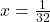## a) 32 x 3 = 3 Find the value of x. b) 78 = 7′ = 76 Find the value of y. It ends in 20 mins :( Thank you

Question

a) 32 x 3 = 3
Find the value of x.
b) 78 = 7′ = 76
Find the value of y.

It ends in 20 mins 🙁
Thank you if you could help!!

in progress 0
4 weeks 2021-08-17T12:25:53+00:00 1 Answers 0 views 0